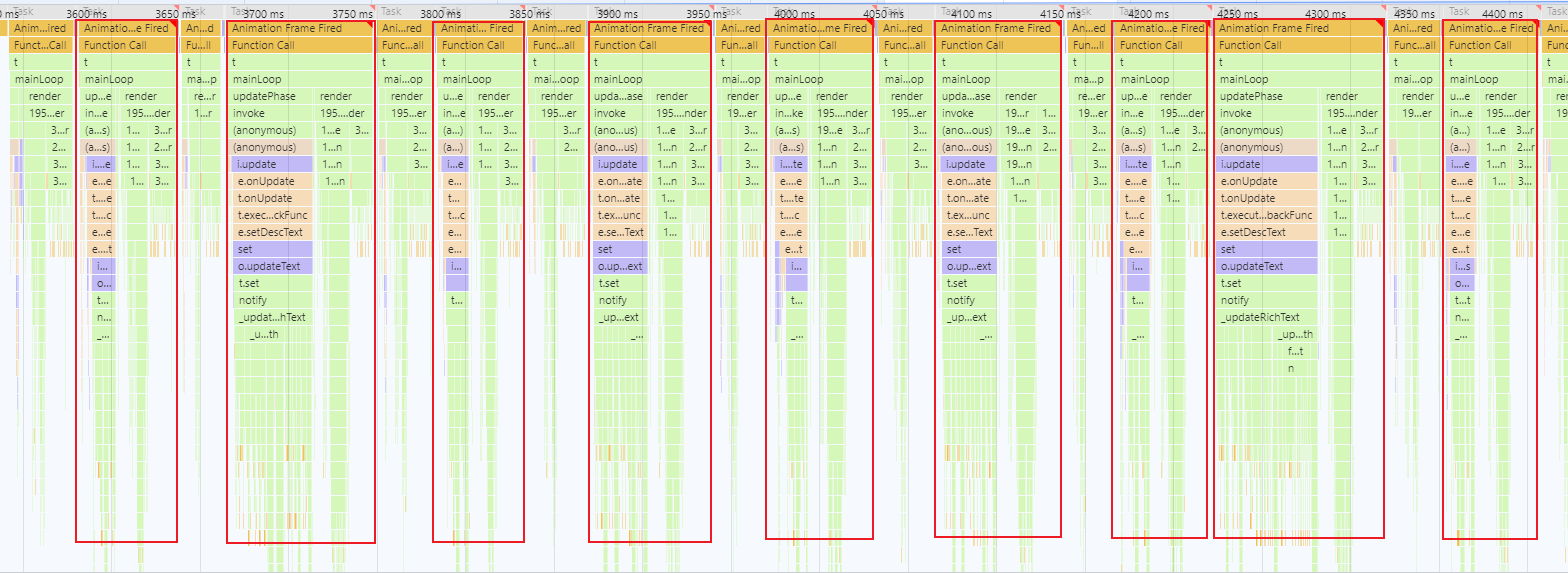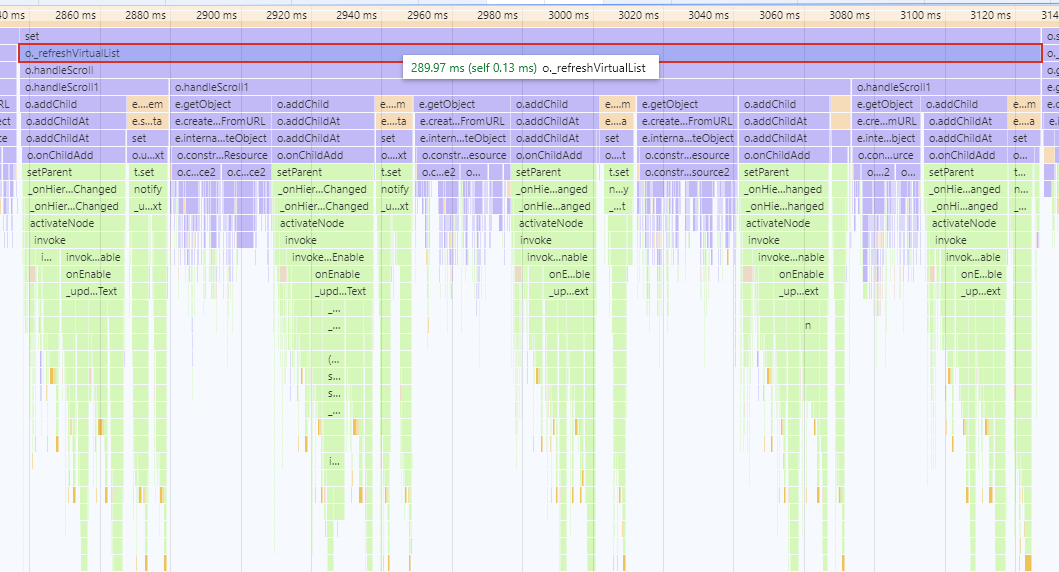# 1. 性能瓶颈分析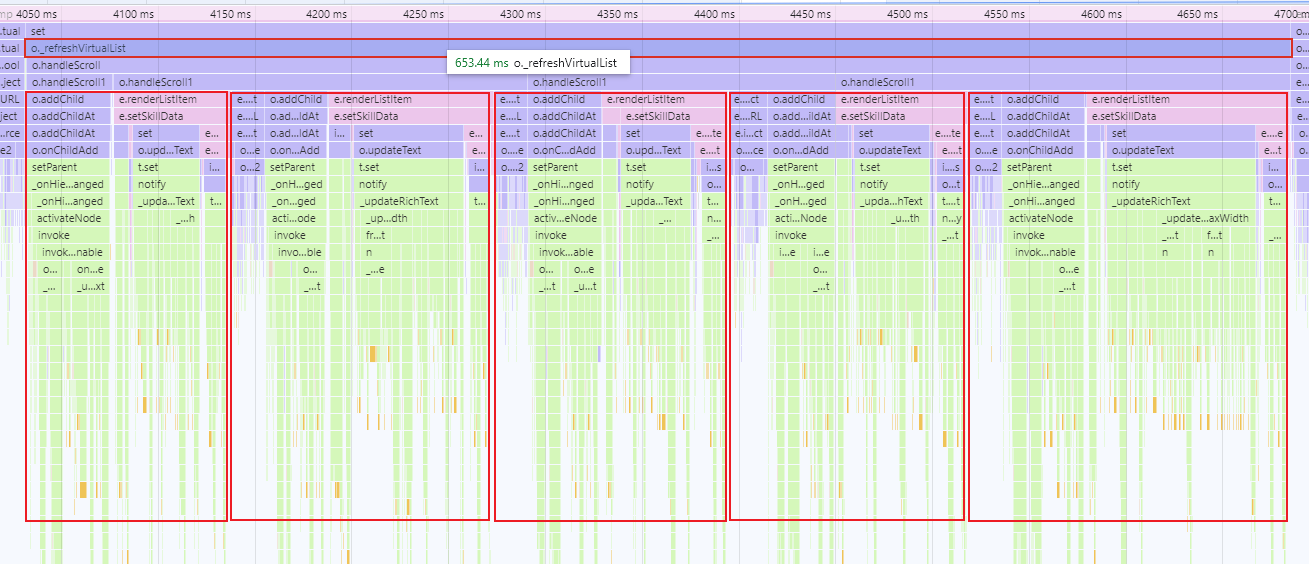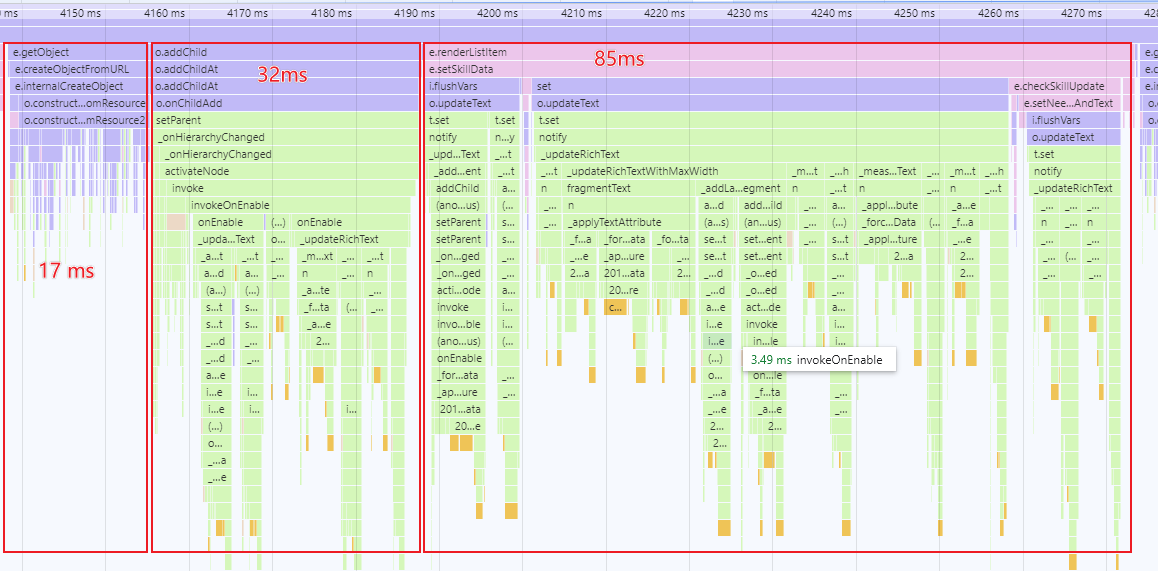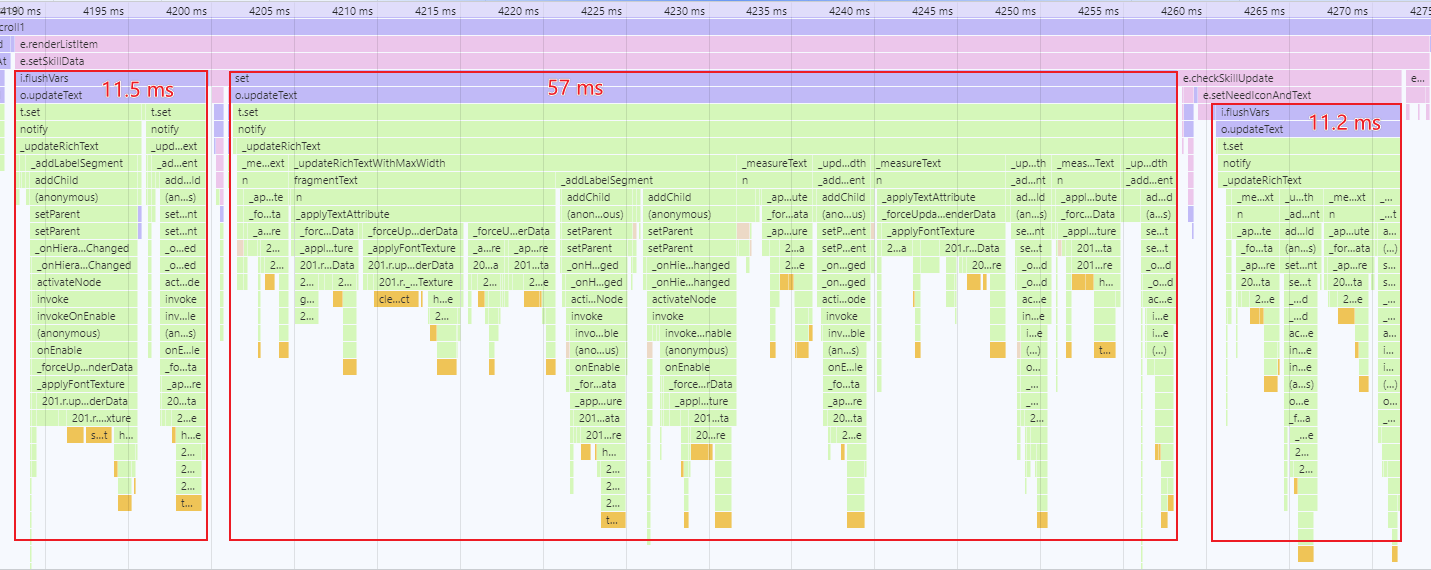# 2. 优化方法

## 2.1 使用普通文本替代富文本

let label: fgui.GRichTextField = this.getchild("label")?.asRichTextField;

let equipName = "屠龙刀";
let equipNum = 1;

// 富文本使用模板
.setVar("equipName", equipName)
.setVar("num", ${equipNum}) .flushVars(); 使用富文本的模板有很大的好处，就是不需要关注具体的文本内容是什么，只需要关注自己需要设置的内容即可。 是一种很好的 UI逻辑 分离的思路，只可惜刷新显示文本时性能消耗有点多。 可以改成普通文本，这样可以节省很多性能。 let label: fgui.GTextField = this.getchild("label")?.asTextField; let userName = "龙傲天"; let equipName = "屠龙刀"; let equipNum = 1; // 普通文本字符串拼接 label.text = 恭喜玩家${userName}获得装备${equipName}x${equipNum}

## 2.2 分帧加载

class renderTaskQueue {
// 单例模式
if (!this.ins) {
}
return this.ins;
}

// 渲染任务队列
private m_queue: Array<Function> = [];

// 添加一个任务到队列里
if (!func || this.m_queue.indexOf(func) < 0) {
this.m_queue.push(func);
}
}

// 从队列中取一个任务并执行
executeOne() {
if (this.m_queue.length > 0) {
let func = this.m_queue.shift();
func && func();
}
}

// 执行队列中所有任务
executeAll() {
this.m_queue.forEach(func => {
func && func();
});
this.m_queue = [];
}
}

// 修改前的 refreshWnd 函数，富文本渲染和图片加载耗时较长
refreshWnd() {
if (this.textField) {
this.textField.text = "这个是普通文本";
}

if (this.richTextField) {
this.richTextField.text = "这个是[color=#d18888]富文本[/color]";
}

}
}

// 优化后的 refreshWnd 函数，将耗时较长的任务拆分出来，放入渲染队列中

setRichTextField() {
if (this.richTextField) {
this.richTextField.text = "这个是[color=#d18888]富文本[/color]";
}
}

}
}

refreshWnd() {
if (this.textField) {
this.textField.text = "这个是普通文本";
}

}

OnUpdate() 中调用 renderTaskQueue 的方法，每帧执行一个任务

OnUpdate() {
// 每帧执行一次 executeOne() 方法，处理一个任务
}

# 3. 优化效果